# Homegrown Effects with the Custom filterThe Custom command enables you to design your own convolution kernel, which is a variety of filter in which neighboring pixels get mixed together. The kernel can be a variation on sharpening, blurring, embossing, or a half- dozen other effects. You create your filter by entering numerical values into a matrix of options.

When you choose Filter Other Custom, Photoshop displays the dialog box shown in Figure 10-1. It sports a 5 5 matrix of text boxes followed by two additional options, Scale and Offset. The matrix options can accept values from negative to positive 999. The Scale value can range from 1 to 9,999, and the Offset value can range from negative to positive 9,999. The dialog box includes Load and Save buttons so that you can load settings from disk and save the current settings for future use.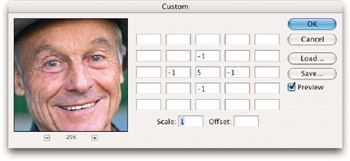Figure 10-1: The Custom dialog box lets you design your own convolution kernel by multiplying the brightness values of pixels.

Like most of Photoshop's filters, the Custom filter also includes a constantly updating preview box, which you'll have lots of time to appreciate if you decide to try your hand at designing your own effects. Select the Preview check box to view the effect of the kernel in the image window as well.

Here's how the filter works: When you press Enter or Return to apply the values in the Custom dialog box to a selection, the filter passes over every pixel in the selection, one at a time. For each pixel being evaluated ” which is called the "PBE" from here on out, just to save ink and paper ” the filter multiplies the PBE's current brightness value by the number in the center option box (the one that contains a 5 in Figure 10-1). To help keep things straight, this value is referred to as the CMV, for central matrix value.

The filter then multiplies the brightness values of the surrounding pixels by the surrounding values in the matrix. For example, Photoshop multiplies the value in the option box just above the CMV by the brightness value of the pixel just above the PBE. It ignores any empty matrix option boxes and the pixels they represent.

Finally, the filter totals the products of the multiplied pixels, divides the sum by the value in the Scale option, and then adds the Offset value to calculate the new brightness of the PBE. It then moves on to the next pixel in the selection and performs the calculation all over again. Figure 10-2 shows a schematic drawing of the process.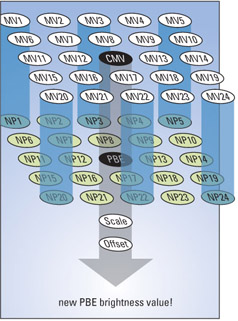Figure 10-2: The Custom filter multiplies each matrix value by the brightness value of the corresponding pixel, adds the products together, divides the sum by the Scale value, adds the Offset value, and applies the result to the pixel being evaluated.

Perhaps seeing all of this spelled out in an equation will help you understand the process; or, you may now know that you have absolutely no interest in using this filter and will be quickly flipping to the latter sections on displacement maps. Either way, here it is. Note that in the following equation, NP stands for neighboring pixel and MV stands for the corresponding matrix value in the Custom dialog box.

New brightness value = ( ( (PBE CMV) + (NP1 MV1) + (NP2 MV2) + . . .) · Scale) + Offset

Luckily, Photoshop calculates the equation without any help from you. All you have to do is punch in the values and see what happens.

Now obviously, if you go around multiplying the brightness value of a pixel too much, you end up making it white. And a filter that turns an image white is pretty much useless. The key, then, is to filter an image and at the same time maintain the original balance of brightness values. To achieve this, just be sure that the sum of all values in the matrix is 1. For example, the default values in the matrix shown back in Figure 10-1 are 5, “1, “1, “1, and “1, which add up to 1. If the sum is greater than 1, use the Scale value to divide the sum down to 1. Figures 10-3 and 10-4 show the results of increasing the CMV to 6, 7, and 8. This raises the sum of the values in the matrix to 2, then 3, and finally 4.

In Figure 10-3, the sum was entered into the Scale option to divide the sum back down to 1 ” any value divided by itself is 1, after all. The result is that Photoshop maintains the original color balance of the image while at the same time filtering it slightly differently. When the Scale value was not raised, the image became progressively lighter, as illustrated in Figure 10-4.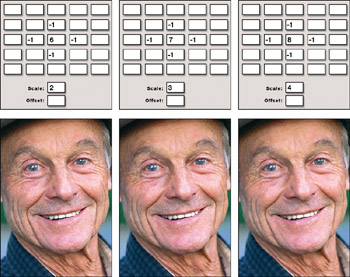Figure 10-3: Raising the Scale value to reflect the sum of the values in the matrix maintains the color balance of the image.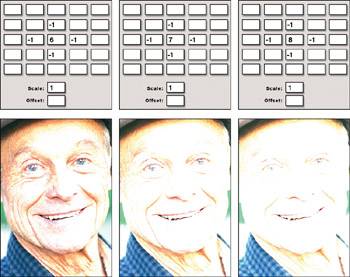Figure 10-4: Raising the sum of the matrix values without counterbalancing it in the Scale option lightens the image.

If the sum is less than 1, increase the CMV until the sum reaches the magic number. For example, in Figure 10-5, the values were lowered to the left of the CMV and then above and to the right of the CMV by 1 apiece to increase the sharpening effect. To ensure that the image did not darken , the CMV was also raised to compensate. When the CMV was not raised, the image turned black.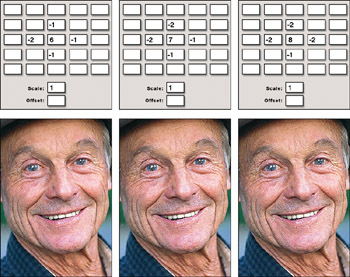Figure 10-5: Raising the CMV to compensate for the lowered values in the matrix maintains the color balance of the image.
 Tip Although a sum of 1 provides the safest and most predictable filtering effects, you can use different sums, such as 0 and 2, to try out more destructive filtering effects. If you do, be sure to raise or lower the Offset value to compensate. For some examples, see the "Non-1 variations" section later in this chapter.

### Applying Custom Values

The following sections show you ways to sharpen, blur, and otherwise filter an image using specific matrix, Scale, and Offset values. Hopefully, by the end of the Custom filter discussions, you will not only know how to repeat the following examples, but also how to apply what you've learned to design special effects of your own.

### Symmetrical effects

Values that are symmetrical both horizontally and vertically surrounding the central matrix value produce sharpen and blur effects:

• Sharpening: A positive CMV surrounded by negative values sharpens an image, as demonstrated in the first example of Figure 10-6. Figures 10-3 through 10-5 also demonstrate varying degrees of sharpening effects.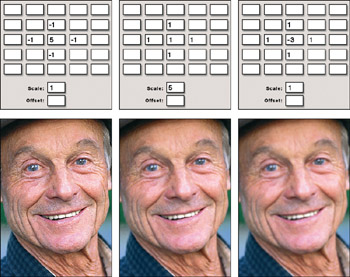Figure 10-6: Symmetrical values can result in sharpening (left), blurring (middle), and edge detection (right) effects.

• Blurring: A positive CMV surrounded by symmetrical positive numbers ” balanced, of course, by a Scale value as explained in the preceding section ” blurs an image, as demonstrated in the second example of Figure 10-6.

• Blurring with edge detection: A negative CMV surrounded by symmetrical positive values blurs an image and adds an element of edge detection, as illustrated in the last example of the figure. These effects are unlike anything provided by Photoshop's standard collection of filters.

### Sharpening

The Custom command provides as many variations on the sharpening theme as the Unsharp Mask filter. In a sense, it provides even more, for whereas the Unsharp Mask filter requires you to sharpen an image inside a Gaussian radius, you get to specify exactly which pixels are taken into account when you use the Custom filter.

To create Unsharp Mask “like effects ” well, more like the Sharpen tool, actually ” enter a large number in the CMV and small values in the surrounding option boxes, as demonstrated in the first two examples of Figure 10-7. To go beyond Unsharp Mask, you can violate the radius of the filter by entering values around the perimeter of the matrix and ignoring options closer to the CMV, as demonstrated in the last example of Figure 10-7.Figure 10-7: To create severe sharpening effects, enter a CMV just large enough to compensate for the negative values in the matrix (first and second examples). To heighten the sharpening effect even further, shift the negative values closer to the perimeter of the matrix (last example).

You can sharpen an image using the Custom dialog box in two basic ways. First, you can enter lots of negative values into the neighboring options in the matrix and then enter a CMV just large enough to yield a sum of 1. This results in radical sharpening effects, as demonstrated in the examples in Figure 10-7.

Second, you can tone down the sharpening by raising the CMV and using the Scale value to divide the sum down to 1. Figure 10-8 shows the results of raising the CMV and Scale values to lessen the impact of sharpening effects typical of those performed in the first two examples of Figure 10-7. Figure 10-9 shows what happens when you apply slightly more adventurous values. Although the arithmetic is a little more involved, the values remain symmetrical and the sums remain 1.Figure 10-8: To sharpen more subtly, increase the central matrix value and then enter the sum into the Scale value.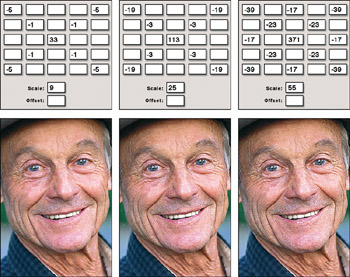Figure 10-9: When you soften the effect of radical sharpening, you create a thicker, higher-contrast effect, much as when raising the Radius value in the Unsharp Mask dialog box.

### Blurring

The philosophy behind blurring is very much the same as that behind sharpening. To produce extreme blurring effects, enter lots of values or high values into the neighboring options in the matrix, enter 1 into the CMV, and then enter the sum into the Scale option. Examples appear in Figure 10-10. To downplay the blurring, raise the CMV and the Scale value by equal amounts. In the last example of Figure 10-10, the same neighboring values were used as in the middle image, but the CMV and the Scale values were increased by 3.Figure 10-10: To create severe blurring effects, enter 1 for the CMV and fill the neighboring options with 1s and 2s (first two images). To blur more subtly, increase the central matrix value and the Scale value by equal amounts (last image).

### Edge detection

Hopefully, this is all beginning to make sense by now. However, in case you're still feeling a little confused , let's go through it one more time in the venue of edge detection. If you really want to see those edges, enter 1s and 2s into the neighboring options in the matrix, and then enter a CMV just small enough ” it's a negative value, after all ” to make the sum 1. The first two examples in Figure 10-11 illustrate .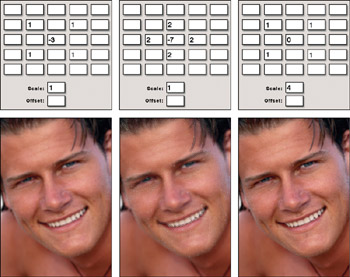Figure 10-11: To create severe edge detection effects, enter a negative CMV just small enough to compensate for the positive values in the matrix first two examples). To blur the edges, increase the central matrix value and then enter the sum into the Scale value (final example).

To lighten the edges and bring out the blur, raise the CMV and enter the resulting sum into the Scale option box. The last example in Figure 10-11 pushes the boundaries between edge detection and a straight blur.

### Non-1 variations

Every image shown in Figures 10-6 through 10-11 is the result of manipulating matrix values and using the Scale option to produce a sum total of 1. Earlier in this chapter, you saw what can happen if you go below 1 (black images) or above 1(white images). But you haven't seen how you can use non-1 totals to produce interesting, if somewhat washed-out, effects.

The key is to raise the Offset value, thereby adding a specified brightness value to each pixel in the image. By doing this, you can offset the lightening or darkening caused by the matrix values to create an image that has half a chance of printing well.

### Lightening overly dark effects

The first image in Figure 10-12 uses nearly the same values used to create the extreme sharpening effect in the last image of Figure 10-7. The only difference is that the CMV is 1 lower (12, down from 13), which in turn lowers the sum total from 1to 0.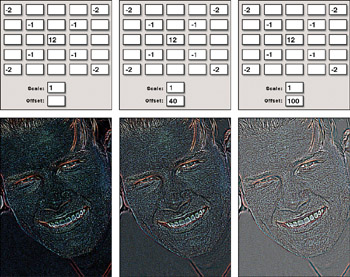Figure 10-12: Three examples of a sharpening kernel with a sum total of 0. The only difference is that the second and third examples were lightened incrementally by entering positive values into the Offset option box.

The result is a dark image with hints of brightness at points of high contrast. The image reads well on-screen, but many of the details are likely to fill in during the printing process. To prevent the image from going too dark, lighten it using the Offset value. Photoshop adds the value to the brightness level of each selected pixel. A brightness value of 255 equals solid white, so you don't need to go too high. As illustrated by the last example in Figure 10-12, an Offset value of 100 is enough to raise most pixels in the image to a medium gray.

### Darkening overly light effects

You also can use the Offset value to darken filtering effects with sum totals greater than 1. The images in Figures 10-13 and 10-14 show sharpening and edge detection effects whose matrix totals add up to 2. On their own, these filters produce effects that are too light. However, as demonstrated in the middle and right examples in the figures, you can darken the effects of the Custom filter to create high-contrast images by entering a negative value into the Offset option box.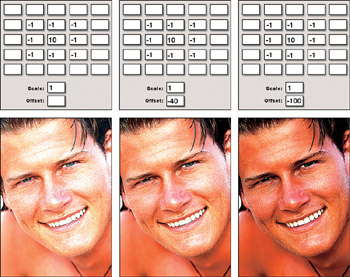Figure 10-13: Three examples of sharpening effects with sum totals of 2. You can darken images incrementally by entering negative values into the Offset option box.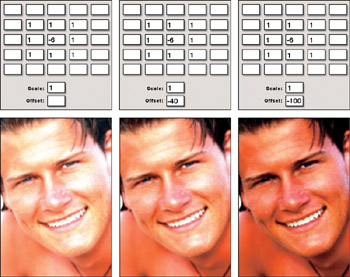Figure 10-14: Three examples of edge detection effects with sum totals of 2, darkened incrementally with progressively lower Offset values.

### Using extreme offsets

If a brightness value of 255 produces solid white and a brightness value of 0 is solid black, why in the world does the Offset value permit any number between negative and positive 9,999, a number 40 times greater than solid white? The answer lies in the fact that the matrix options can force the Custom filter to calculate brightness values much darker than black and much lighter than white. Therefore, you can use a very high or very low Offset value to boost the brightness of an image in which all pixels are well below black or diminish the brightness when all pixels are way beyond white.

Figure 10-15 shows exaggerated versions of the sharpening, blurring, and edge detection effects. The sum totals of the matrices (when divided by the Scale values) are “42, 53, and 42, respectively. Without some help from the Offset value, each of these filters would turn every pixel in the image black (in the case of the sharpening effect) or white (blurring and edge detection). But as demonstrated in the figure, using enormous Offset numbers brings out those few brightness values that remain. The images are so polarized that there's little difference between the three effects, the first image being an inverted version of the last. The second row shows the results of choosing Edit Fade Custom immediately after applying the Custom filter and selecting the Luminosity blend mode.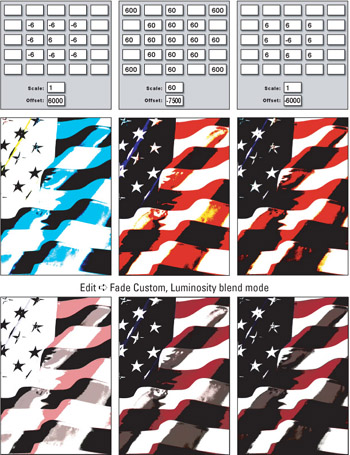Figure 10-15: You can create high-contrast effects by exaggerating all values in the matrix and then compensating with a very high or very low Offset value (top row). When you back off the effect using Edit Fade (bottom row), these dramatic effects become interesting indeed.

### Other custom effects

By now, you may see what a very cool and flexible tool the Custom filter can be. At the very least, you now have a better idea of how it works, and if you tried any of the settings shown, you have seen it go to work on your images. In truth, another 20 or 30 pages that could easily have been devoted to the myriad ways the Custom filter can be used, and even that wouldn't truly do the topic justice .

Given that the demonstrations thus far have been fairly tame ” showing you how to use the Custom filter to achieve effects that can also be obtained through other filters, either alone or in combination ” you may be wondering what happens if you just go absolutely berserk, in a crazed computer-geek sort of way, and start entering matrix values in random, arbitrary arrangements. The answer is that as long as you maintain a sum total of 1, you can achieve some pretty interesting and even usable effects. Many of these effects will be simple variations on blurring, sharpening, and edge detection, which are covered in upcoming sections of this chapter.

### Directional blurs

Figure 10-16 shows examples of entering positive matrix values all in one row, all in a column, or in opposite quadrants. As you can see, as long as you maintain uniformly positive values, you get a blurring effect. However, by keeping the values lowest in the center and highest toward the edges and corners, you can create directional blurs. The first example resembles a slight horizontal motion blur, the second looks like a slight vertical motion blur, and the last example looks like it's vibrating horizontally and vertically.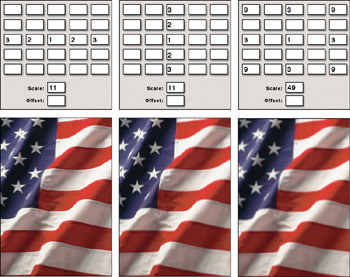Figure 10-16: Enter positive matrix values in a horizontal formation (left) or vertical formation (middle) to create slight motion blurs. By positioning positive values in opposite corners of the matrix, you create a subtle vibration effect (right) as the content is blurred in two directions, as though it's being shaken in place.

### Embossing

So far, you aren't going very nuts, are you? Despite their unusual formations, the matrix values in Figures 10-16 still manage to maintain symmetry. Well, now it's time to lose the symmetry, which typically results in an embossing effect.

Figure 10-17 shows three variations on embossing, all of which involve positive and negative matrix values positioned on opposite sides of the CMV. (The CMV happens to be positive merely to maintain a sum total of 1.)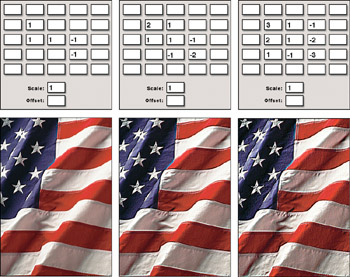Figure 10-17: You can create embossing effects by distributing positive and negative values on opposite sides of the central matrix value.

This type of embossing has no hard-and-fast light source, but you might imagine that the light comes from the general direction of the positive values. Therefore, when the positive and negative values are swapped throughout the matrix (all except the CMV), an underlighting effect is achieved, as demonstrated by the images in Figure 10-18.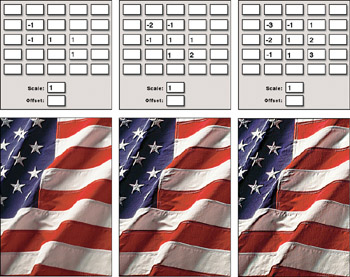Figure 10-18: Change the location of positive and negative matrix values to change the general direction of the light source.

In truth, it's not so much a lighting difference as a difference in edge enhancement. White pixels collect on the side of an edge represented by positive values in the matrix; black pixels collect on the negative-value side. So when the locations of positive and negative values are swapped between Figures 10-17 and 10-18, the distribution of white and black pixels in the filtered images is changed as well.

Embossing is the loosest of the Custom filter effects. As long as you position positive and negative values on opposite sides of the CMV, you can distribute the values in almost any way you see fit. Figure 10-19 demonstrates three entirely arbitrary arrangements of values in the Custom matrix, downplayed by raising the CMV and entering the sum of the matrix values in the Scale option box. Notice, too, that unlike Filter Stylize Emboss, which sacrifices color and changes low-contrast areas to gray, the Custom filter preserves the natural colors in the image.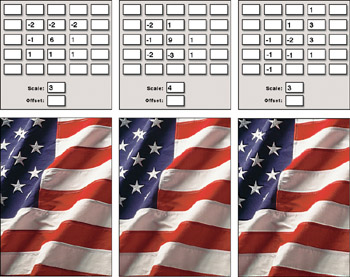Figure 10-19: You can create whole libraries of embossing effects by experimenting with different combinations of positive and negative values. To emboss more subtly, increase the central matrix value and the Scale values by equal amounts.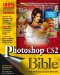Photoshop CS2 Bible
ISBN: 0764589725
EAN: 2147483647
Year: 2003
Pages: 95